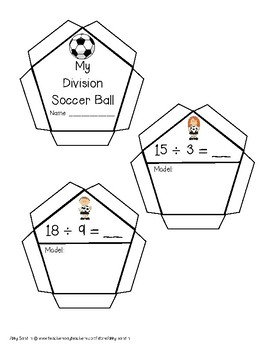# Interactive DivisionSoccer Ball Craftivity {3.OA.A.1, 3.OA.A.3, 3.OA.C.7}Subject
Resource Type
File Type

PDF

(1 MB|11 pages)
Product Rating
Standards
• Product Description
• StandardsNEW
Reinforce division concepts with this interactive and engaging "soccer ball." Students will solve 12 soccer themed division problems by drawing a model and then use the pieces to construct a "soccer ball!" Constructing the soccer ball is a mini logic puzzle in itself!

Included:
Title Page
Thank you page
How to Use This
12 Division Problems using Models

Happy learning!
Abby Sandlin

www.teacherspayteachers.com/store/abby-sandlin
Multiply or divide to solve word problems involving multiplicative comparison, e.g., by using drawings and equations with a symbol for the unknown number to represent the problem, distinguishing multiplicative comparison from additive comparison.
Interpret a multiplication equation as a comparison, e.g., interpret 35 = 5 × 7 as a statement that 35 is 5 times as many as 7 and 7 times as many as 5. Represent verbal statements of multiplicative comparisons as multiplication equations.
Fluently multiply and divide within 100, using strategies such as the relationship between multiplication and division (e.g., knowing that 8 × 5 = 40, one knows 40 ÷ 5 = 8) or properties of operations. By the end of Grade 3, know from memory all products of two one-digit numbers.
Use multiplication and division within 100 to solve word problems in situations involving equal groups, arrays, and measurement quantities, e.g., by using drawings and equations with a symbol for the unknown number to represent the problem.
Interpret products of whole numbers, e.g., interpret 5 × 7 as the total number of objects in 5 groups of 7 objects each. For example, describe a context in which a total number of objects can be expressed as 5 × 7.
Total Pages
11 pages
Included
Teaching Duration
N/A
Report this Resource to TpT
Reported resources will be reviewed by our team. Report this resource to let us know if this resource violates TpT’s content guidelines.